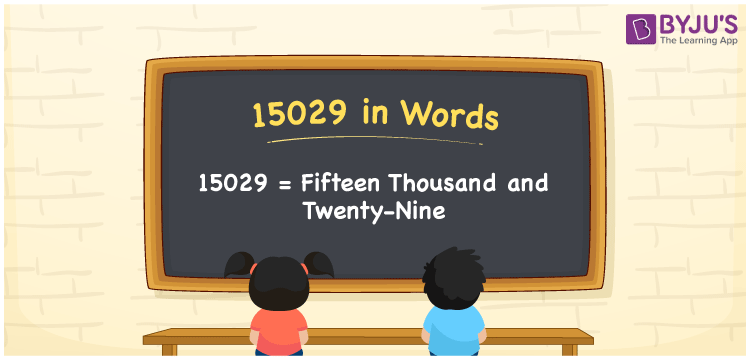# 15029 in Words

15029 in words is written as “fifteen thousand and twenty-nine”. 15029 is a five-digit number and it is also considered to be a cardinal number. To write any numbers in words, we usually prefer the place value system. Now, let us have a look at the process of writing the number 15029 in words with a complete explanation.

 15029 in Words: Fifteen Thousand and Twenty-nine. Fifteen Thousand and Twenty-nine in Numerical Form: 15029.

## 15029 in English Words## How to Write 15029 in Words?

Go through the place value table for the numeral 15029.

 Ten-thousands Thousands Hundreds Tens Ones 1 5 0 2 9

The expanded form of 15029 is as follows:

= 1 × Ten thousand + 5 × Thousand + 0 × Hundred + 2 × Ten + 9 × One

= 1 × 10000 + 5 × 1000 + 0 × 100 + 2 × 10 + 9 × 1

= 10000 + 5000 + 20 + 9

= 15029

= fifteen thousand and twenty-nine

Hence, 15029 in words is fifteen thousand and twenty-nine.

15029 in words – Fifteen thousand and twenty-nine

Is 15029 an odd number? – Yes

Is 15029 an even number? – No

Is 15029 a perfect square number? – No

Is 15029 a perfect cube number? – No

Is 15029 a prime number? – No

Is 15029 a composite number? – Yes

## Frequently Asked Questions on 15029 in Words

Q1

### How to write 15029 in English words?

15029 in words is fifteen thousand and twenty-nine.

Q2

### Simplify 15000 + 29, and express it in words.

Simplifying 15000 + 29, we get 15029. Hence, 15029 in words is fifteen thousand and twenty-nine.

Q3

### Is 15029 an odd number?

Yes, 15029 is an odd number.